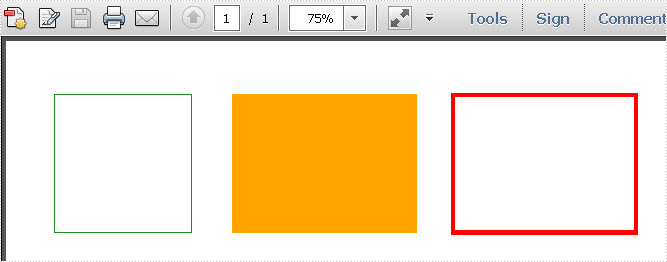# Draw Rectangles in PDF in C#, VB.NET

In Euclidean plane geometry, a rectangle is any quadrilateral with four right angles. The term "oblong" is occasionally used to refer to a non-square rectangle. A rectangle with vertices ABCD would be denoted as ABCD. It’s simple for people to draw rectangles in paper. While how about drawing rectangles in PDF document? This section will show you the exact answer. This section will introduce a solution to draw rectangles and set the size and position of rectangles in PDF via a .NET PDF component Spire.PDF for .NET with C#, VB.NET.

In Spire.PDF, there are two classes: Spire.Pdf.Graphics.PdfPen and Spire.Pdf.Granphics.PdfBrush. By using the first class, we can set the color and decide the outline of the PDF rectangle. While the second class can quickly help us fill the rectangles with a color we want. Now let us see this method: Spire.Pdf.PdfPageBase.Canvas.DrawRectangle(PdfPen pen, RectangleF rectangle); There are two parameters passed. One is the PdfPen which I referred above. The other represents the location and size of a rectangle. By calling this method, we can draw rectangles and set their size and position very quickly. Now let us view the rectangles as below picture:Here we can download Spire.PDF for .NET and install it on system. After adding Spire.Pdf dll, we can draw rectangle in our PDF document as below code:

[C#]
```using System.Drawing;
using Spire.Pdf;
using Spire.Pdf.Graphics;

namespace PDF_rectangles
{
class Program
{
static void Main(string[] args)
{
//create a PDF
PdfDocument pdfDoc = new PdfDocument();
//save graphics state
PdfGraphicsState state = page.Canvas.Save();
//draw rectangles
PdfPen pen = new PdfPen(Color.ForestGreen, 0.1f);
PdfPen pen1 = new PdfPen(Color.Red, 3f);
PdfBrush brush = new PdfSolidBrush(Color.Orange);
page.Canvas.DrawRectangle(pen, new Rectangle(new Point(2, 7), new Size(120, 120)));
page.Canvas.DrawRectangle(pen1, new Rectangle(new Point(350, 7), new Size(160, 120)));
page.Canvas.DrawRectangle(brush, new RectangleF(new Point(158, 7), new SizeF(160, 120)));
//restor graphics
page.Canvas.Restore(state);
pdfDoc.SaveToFile("Rectangles.pdf");
System.Diagnostics.Process.Start("Rectangles.pdf");
}
}
}
```
[VB.NET]
```Imports System.Drawing
Imports Spire.Pdf
Imports Spire.Pdf.Graphics

Namespace PDF_rectangles
Class Program
Private Shared Sub Main(args As String())
'create a PDF
Dim pdfDoc As New PdfDocument()
Dim page As PdfPageBase = pdfDoc.Pages.Add()
'save graphics state
Dim state As PdfGraphicsState = page.Canvas.Save()
'draw rectangles
Dim pen As New PdfPen(Color.ForestGreen, 0.1F)
Dim pen1 As New PdfPen(Color.Red, 3F)
Dim brush As PdfBrush = New PdfSolidBrush(Color.Orange)
page.Canvas.DrawRectangle(pen, New Rectangle(New Point(2, 7), New Size(120, 120)))
page.Canvas.DrawRectangle(pen1, New Rectangle(New Point(350, 7), New Size(160, 120)))
page.Canvas.DrawRectangle(brush, New RectangleF(New Point(158, 7), New SizeF(160, 120)))
'restor graphics
page.Canvas.Restore(state)
pdfDoc.SaveToFile("Rectangles.pdf")
System.Diagnostics.Process.Start("Rectangles.pdf")
End Sub
End Class
End Namespace
```

Spire.PDF for .NET is a .NET PDF component that can draw different kinds of shapes in PDF document such as Circles, Arcs. Ellipse and Five-pointed Star.